Скачать презентацию Coulometric Methods A Introduction 1 Coulometry

f4180852d4aa730cee9878998ce30a96.ppt

• Количество слайдов: 10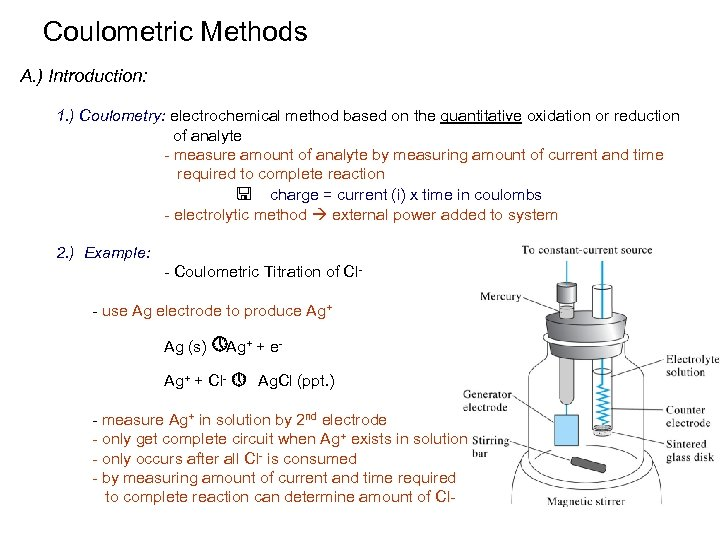Coulometric Methods A. ) Introduction: 1. ) Coulometry: electrochemical method based on the quantitative oxidation or reduction of analyte - measure amount of analyte by measuring amount of current and time required to complete reaction < charge = current (i) x time in coulombs - electrolytic method external power added to system 2. ) Example: - Coulometric Titration of Cl- use Ag electrode to produce Ag+ Ag (s) » Ag+ + e. Ag+ + Cl- » Ag. Cl (ppt. ) - measure Ag+ in solution by 2 nd electrode - only get complete circuit when Ag+ exists in solution - only occurs after all Cl- is consumed - by measuring amount of current and time required to complete reaction can determine amount of Cl-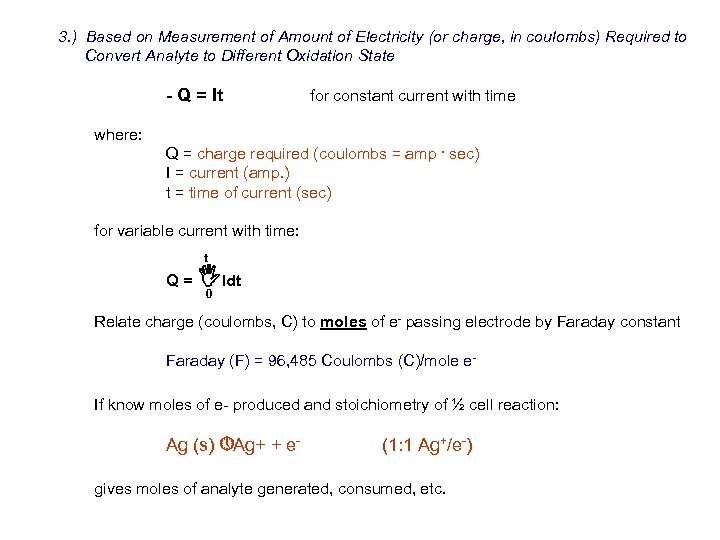3. ) Based on Measurement of Amount of Electricity (or charge, in coulombs) Required to Convert Analyte to Different Oxidation State - Q = It where: for constant current with time Q = charge required (coulombs = amp. sec) I = current (amp. ) t = time of current (sec) for variable current with time: t Q= IIdt 0 Relate charge (coulombs, C) to moles of e- passing electrode by Faraday constant Faraday (F) = 96, 485 Coulombs (C)/mole e. If know moles of e- produced and stoichiometry of ½ cell reaction: Ag (s) » Ag+ + e- (1: 1 Ag+/e-) gives moles of analyte generated, consumed, etc.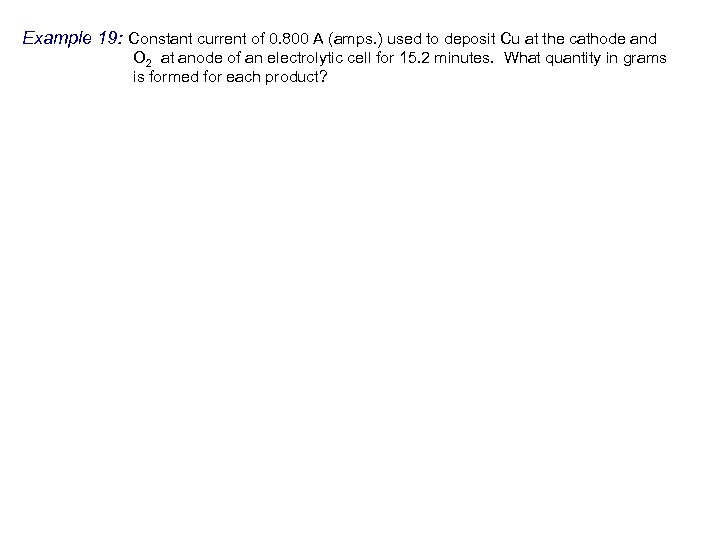Example 19: Constant current of 0. 800 A (amps. ) used to deposit Cu at the cathode and O 2 at anode of an electrolytic cell for 15. 2 minutes. What quantity in grams is formed for each product?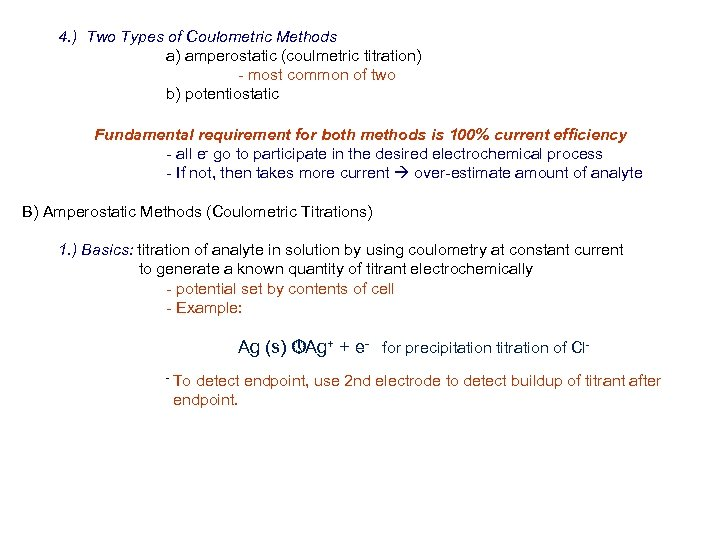4. ) Two Types of Coulometric Methods a) amperostatic (coulmetric titration) - most common of two b) potentiostatic Fundamental requirement for both methods is 100% current efficiency - all e- go to participate in the desired electrochemical process - If not, then takes more current over-estimate amount of analyte B) Amperostatic Methods (Coulometric Titrations) 1. ) Basics: titration of analyte in solution by using coulometry at constant current to generate a known quantity of titrant electrochemically - potential set by contents of cell - Example: Ag (s) » Ag+ + e- for precipitation titration of Cl- To detect endpoint, use 2 nd electrode to detect buildup of titrant after endpoint.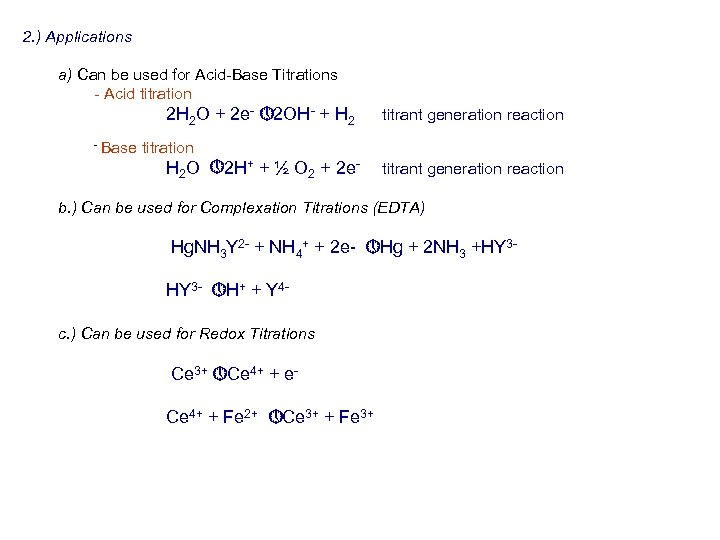2. ) Applications a) Can be used for Acid-Base Titrations - Acid titration 2 H 2 O + 2 e- » 2 OH- + H 2 - Base titrant generation reaction titration H 2 O » 2 H+ + ½ O 2 + 2 e- titrant generation reaction b. ) Can be used for Complexation Titrations (EDTA) Hg. NH 3 Y 2 - + NH 4+ + 2 e- » Hg + 2 NH 3 +HY 3 - » H+ + Y 4 c. ) Can be used for Redox Titrations Ce 3+ » Ce 4+ + e. Ce 4+ + Fe 2+ » Ce 3+ + Fe 3+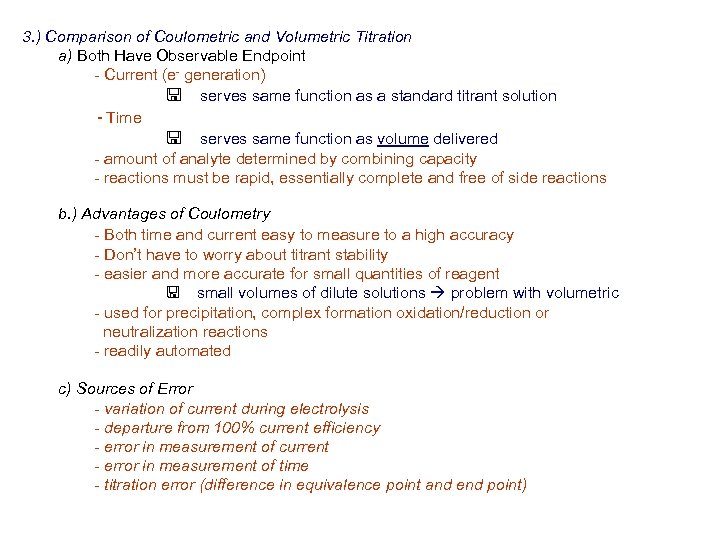3. ) Comparison of Coulometric and Volumetric Titration a) Both Have Observable Endpoint - Current (e- generation) < serves same function as a standard titrant solution - Time < serves same function as volume delivered - amount of analyte determined by combining capacity - reactions must be rapid, essentially complete and free of side reactions b. ) Advantages of Coulometry - Both time and current easy to measure to a high accuracy - Don’t have to worry about titrant stability - easier and more accurate for small quantities of reagent < small volumes of dilute solutions problem with volumetric - used for precipitation, complex formation oxidation/reduction or neutralization reactions - readily automated c) Sources of Error - variation of current during electrolysis - departure from 100% current efficiency - error in measurement of current - error in measurement of time - titration error (difference in equivalence point and end point)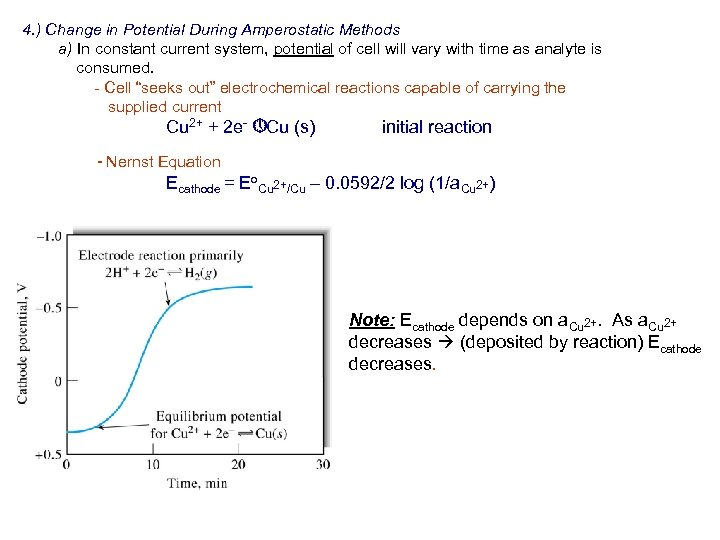4. ) Change in Potential During Amperostatic Methods a) In constant current system, potential of cell will vary with time as analyte is consumed. - Cell “seeks out” electrochemical reactions capable of carrying the supplied current Cu 2+ + 2 e- » Cu (s) initial reaction - Nernst Equation Ecathode = Eo. Cu 2+/Cu – 0. 0592/2 log (1/a. Cu 2+) Note: Ecathode depends on a. Cu 2+. As a. Cu 2+ decreases (deposited by reaction) Ecathode decreases.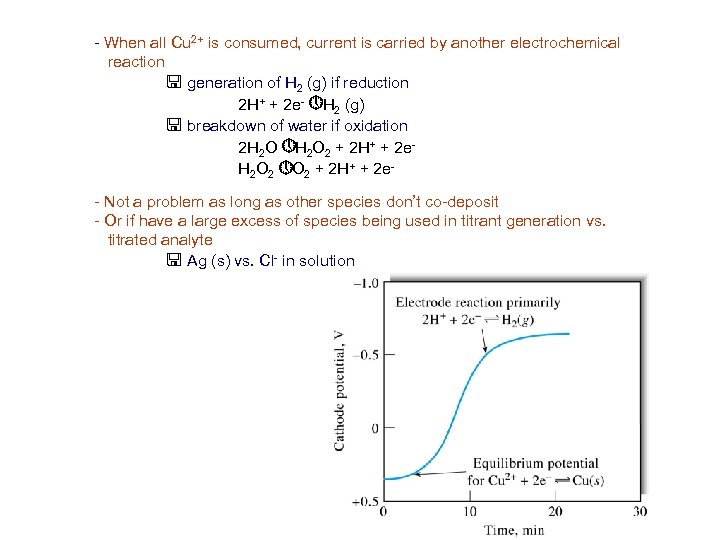- When all Cu 2+ is consumed, current is carried by another electrochemical reaction < generation of H 2 (g) if reduction 2 H+ + 2 e- » H 2 (g) < breakdown of water if oxidation 2 H 2 O » H 2 O 2 + 2 H+ + 2 e. H 2 O 2 » O 2 + 2 H+ + 2 e- Not a problem as long as other species don’t co-deposit - Or if have a large excess of species being used in titrant generation vs. titrated analyte < Ag (s) vs. Cl- in solution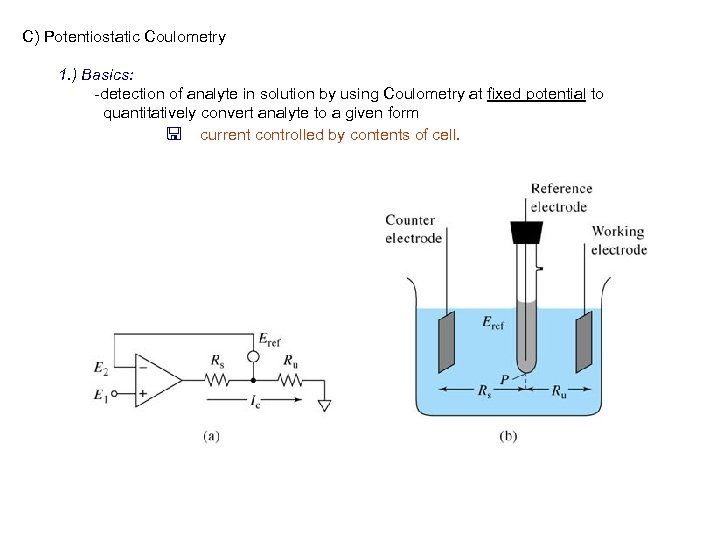C) Potentiostatic Coulometry 1. ) Basics: -detection of analyte in solution by using Coulometry at fixed potential to quantitatively convert analyte to a given form < current controlled by contents of cell.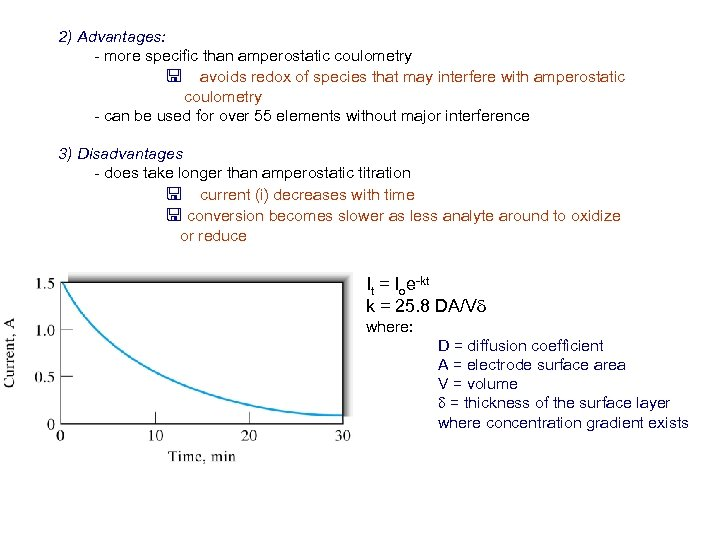2) Advantages: - more specific than amperostatic coulometry < avoids redox of species that may interfere with amperostatic coulometry - can be used for over 55 elements without major interference 3) Disadvantages - does take longer than amperostatic titration < current (i) decreases with time < conversion becomes slower as less analyte around to oxidize or reduce It = Ioe-kt k = 25. 8 DA/Vd where: D = diffusion coefficient A = electrode surface area V = volume d = thickness of the surface layer where concentration gradient exists